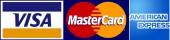# ETNA PRESTIGE TECHNOLOGY

www.etnapt.com > ATU/BTU Calculator

# ATU/BTU Calculator

HOW TO CALCULATE HVAC UNIT SIZE

1. 1. FIGURE OUT THE SQUARE FOOTAGE OF SPACE THAT YOU NEED TO HEAT AND COOL. MEASURE THE ROOM’S LENGTH AND WIDTH. MULTIPLY THESE TOGETHER TO GET THE SQUARE FOOTAGE
2. 2. CONTINUE MEASURING EACH ROOM AND ADD THE SQUARE FOOTAGES TOGETHER. AS A GENERAL RULE, YOU’LL WANT ONE CFM(CUBIC FEET PER MINUTE) PER SQUARE FOOT. THE EXCEPTION TO THIS IS IF YOU HAVE AN AREA WITH A LOT OF WINDOWS. YOU’LL THEN WANT TWO CFM PER SQUARE FOOT.
3. 3. CONVERT THE CFM’S INTO TONS BY DIVIDING BY 400. FOR EXAMPLE, SAY YOUR TOTAL SQUARE FOOTAGE IS 2000 AND YOU DETERMINED YOU NEED 2000CFM. TAKE 2000 AND DIVIDE BY 400. FOR THIS EXAMPLE YOU NEED A FIVE TON CONDITIONING UNIT.

ATU(AVERAGE TONS PER UNIT) = CFM/400= 2000/400=5 TON

HOW TO CALCULATE BTU

1. BTU(BRITISH THERMAL UNITS) NEEDEN FOR YOUR FURNACE. THIS IS DONE BY TAKING YOUR CFM AND DIVIDING BY 150 AND THEN MULTIPLYING THAT NUMBER BY 10.000. FOR INSTANCE, FROM THE EXAMPLE IN STEP 3 YOU NEED 2000 CFM.     2000/150= 13.1/3

THIS NUMBER MULTIPLIED BY 10 000= 133.333 BTU

YOU WOULD INSTALL A FURNACE CLOSE TO THAT OUTPUT OF BTU.

Determine the size of your HVAC unit with our

### ATU/BTU Calculatorwhat is this?

Area Sq. Footage:
Area has a lot of windows:

ATU:
BTU:

### Let's socialize

#### We accept major credit cards